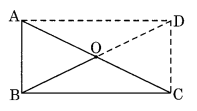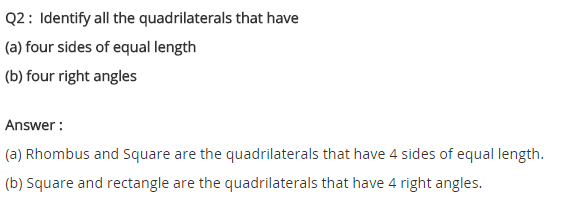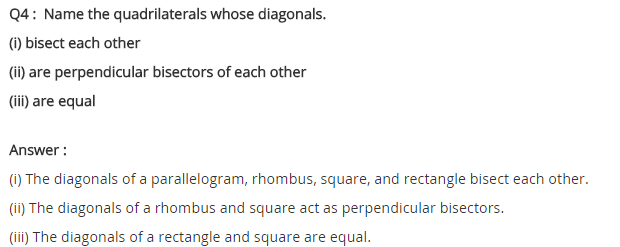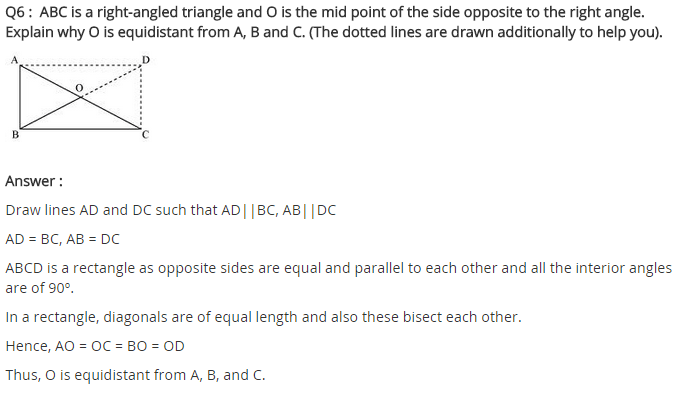# NCERT Solutions for Class 8 Maths Chapter 3 Understanding Quadrilaterals Ex 3.4

NCERT Solutions for Class 8 Maths Chapter 3 Understanding Quadrilaterals Exercise 3.4

Ex 3.4 Class 8 Maths Question 1.
State whether True or False.
(a) All rectangles are squares.
(b) All rhombuses are parallelograms.
(c) All squares are rhombuses and also rectangles.
(d) All squares are not parallelograms.
(e) All kites are rhombuses.
(f) All rhombuses are kites.
(g) All parallelograms are trapeziums.
(h) All squares are trapeziums.
Solution:
(a) False
(b) True
(c) True
(d) False
(e) False
(f) True
(g) True
(h) True

Ex 3.4 Class 8 Maths Question 2.
Identify all the quadrilaterals that have
(a) four sides of equal length
(b) four right angles
Solution:
(a) Squares and rhombuses.
(b) Rectangles and squares.

Ex 3.4 Class 8 Maths Question 3.
Explain how a square is
(ii) a parallelogram
(iii) a rhombus
(iv) a rectangle
Solution:
(i) Square is a quadrilateral because it is closed with four line segments.
(ii) Square is a parallelogram due to the following properties:
(a) Opposite sides are equal and parallel.
(b) Opposite angles are equal.
(iii) Square is a rhombus because its all sides are equal and opposite sides are parallel.
(iv) Square is a rectangle because its opposite sides are equal and has equal diagonal.

Ex 3.4 Class 8 Maths Question 4.
(i) bisect each other
(ii) are perpendicular bisectors of each other
(iii) are equal
Solution:
(i) Parallelogram, rectangle, square and rhombus
(ii) Square and rhombus
(iii) Rectangle and square

Ex 3.4 Class 8 Maths Question 5.
Explain why a rectangle is a convex quadrilateral.
Solution:
In a rectangle, both of its diagonal lie in its interior. Hence, it is a convex quadrilateral.

Ex 3.4 Class 8 Maths Question 6.
ABC is a right-angled triangle and O is the midpoint of the side opposite to the right angle. Explain why O is equidistant from A, B and C. (The dotted lines are drawn additionally to help you).Solution:
Since the right-angled triangle ABC makes a rectangle ABCD by the dotted lines.
Therefore OA = OB = OC = OD [Diagonals of a rectangle are equal and bisect each other]
Hence, O is equidistant from A, B and C.+